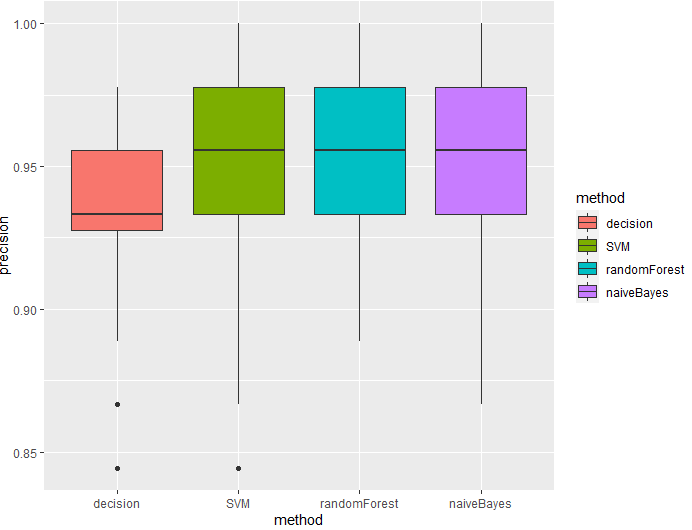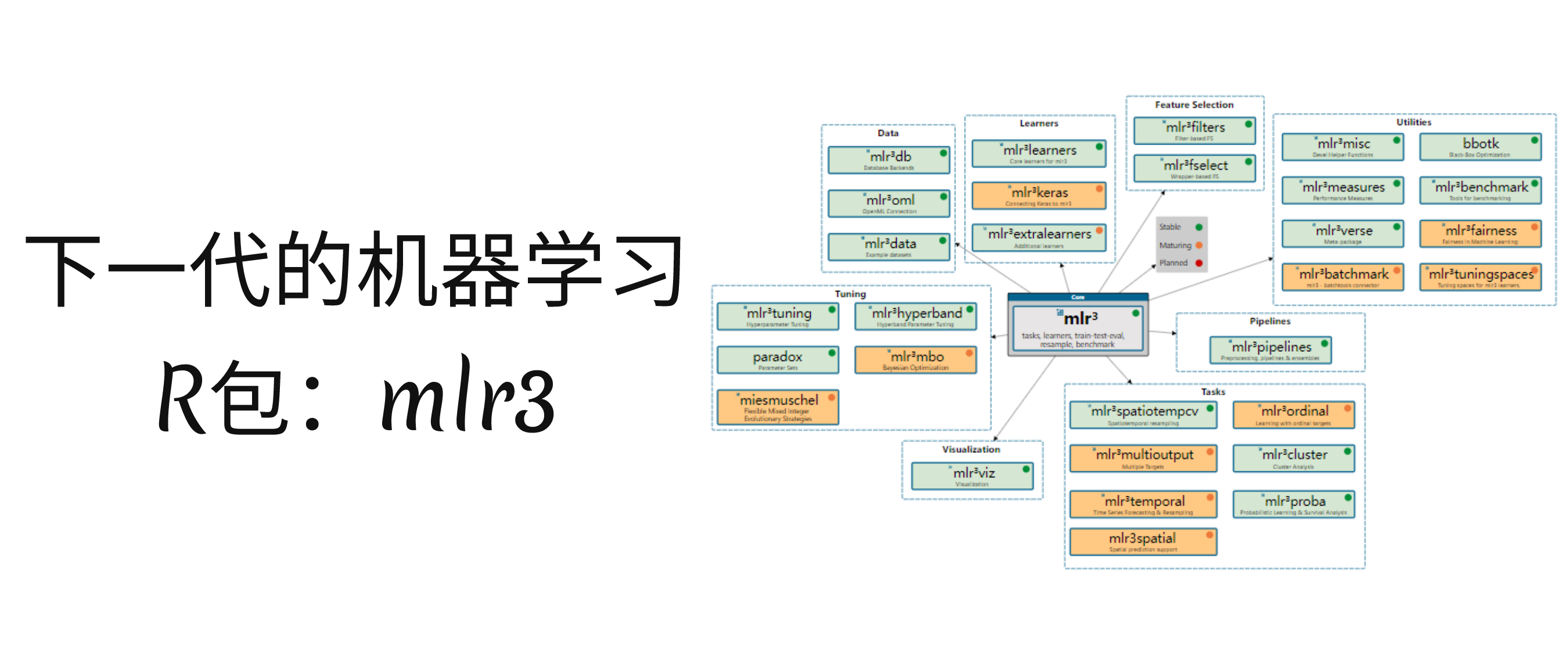• R语言机器学习模型-xgboost回归代码
2022-03-06 10:08:40

采用xgboost包构建xgboost模型有一个比较棘手问题在于构建xgb.DMatrix数据，同时为了应用提前终止策略需要构建watchlist，以下代码从数据准备部分开始对上面两部分内容均有覆盖，大家可以参考使用。

# 数据准备colnames(boston)# 训练集dvfunc <- dummyVars(~., data = data_train[, 1:13], fullRank = T)data_trainx <- predict(dvfunc, newdata = data_train[, 1:13])data_trainy <- data_train$medv# 验证集data_validx <- predict(dvfunc, newdata = data_valid[, 1:13])data_validy <- data_valid$medv# 测试集data_testx <- predict(dvfunc, newdata = data_test[, 1:13])data_testy <- data_test$medv# 构建xgb.DMatrix格式数据dtrain <- xgb.DMatrix(data = data_trainx, label = data_trainy)dvalid <- xgb.DMatrix(data = data_validx, label = data_validy)dtest <- xgb.DMatrix(data = data_testx, label = data_回归 更多相关内容 • 简单介绍一下R语言的几个分类算法便于以后查找 # install.packages("DMwR") # install.packages("kernlab") # install.packages("Hmisc") # install.packages("corrplot") # install.packages("mlogit") # install.... 简单介绍一下R语言的几个分类算法便于以后查找 # install.packages("DMwR") # install.packages("kernlab") # install.packages("Hmisc") # install.packages("corrplot") # install.packages("mlogit") # install.packages("randomForest") # install.packages("e1071") # 决策树方法 set.seed(42) #加载决策树包 library(rpart) data("iris") #抽取用于建模的数据，70%的数据集进行建模 ind <- sample(1:150, 105) #构建决策树模型，因为是对分类进行建模，class m <- rpart(formula = Species~., data = iris[ind,],method = "class") summary(m) print(m) #绘制决策树图像，看出决策的过程和规则 rpart.plot::rpart.plot(m) pre <- predict(m, iris[-ind, -5], type = "class") table(pre, iris$Species[-ind])
sum(pre == iris$Species[-ind])/length(pre) # 尝试一百次决策树 result3 <- 0 for(i in 1:100) { ind <- sample(1:150, 105) m <- rpart(formula = Species~., data = iris[ind,], method = "class") pre <- predict(m, iris[-ind, -5], type = "class") table(pre, iris$Species[-ind])
result3[i] <- sum(pre == iris$Species[-ind])/length(pre) } mean(result3) table(pre, iris[-ind, 5]) # 支持向量机 library(kernlab) result4 <- 0 for(i in 1:100) { ind <- sample(1:150, 105) k <- ksvm(x = Species~., iris[ind,], C = 10, type = "C-svc") #, C = 10, type = "C-svc" # type类型可以选择分类或者回归 pre <- predict(k, iris[-ind,]) result4[i] <- sum(pre == iris$Species[-ind])/length(pre)
}
mean(result4)
table(pre, iris[-ind, 5])

# 随机森林
library(randomForest)
result5 <- 0
for(i in 1:100)
{
ind <- sample(1:150,105)
k <- randomForest(Species ~ ., data = iris[ind, ]) #, ntree = 100, proximity = T
pre <- predict(k, iris[-ind, ])
result5[i] <- sum(pre == iris$Species[-ind])/length(pre) } mean(result5) table(pre, iris[-ind, 5]) #朴素贝叶斯 library(e1071) result6 <- 0 for(i in 1:100) { ind <- sample(1:150, 105) k <- naiveBayes(Species ~ ., data = iris[ind, ]) pre <- predict(k, iris[-ind, ]) result6[i] <- sum(pre == iris$Species[-ind])/length(pre)
}
mean(result6)
table(pre, iris[-ind, 5])

#模型之间的比较
table(pre,iris[-ind, 5])
result <- data.frame(precision =
c(result3, result4, result5, result6),
method = gl(n = 4, k = 100, labels =
c("decision",
"SVM", "randomForest", "naiveBayes")))

ggplot(data = result, mapping =
aes(y = precision, x = method, fill = method))+
geom_boxplot()展开全文决策树
• r语言随机森林包，随机森林是基于决策树的一种机器学习语言。用于医学预测，生态发展预测，且预测精度高。 r语言随机森林包，随机森林是基于决策树的一种机器学习语言。用于医学预测，生态发展预测，且预测精度高。随机森林
• mlr3包和其扩展包为R语言提供了一个统一的、面向对象的、可扩展的机器学习框架，可用于回归、分类、生存分析和其他机器学习任务。mlr3并没有引入新的机器学习算法，而是为R中的机器学习R包通过了统一的接口，通过这...

获取更多R语言和生信知识，请关注公众号：医学和生信笔记。
公众号后台回复R语言，即可获得海量学习资料！

## mlr3简介

mlr3包和其扩展包为R语言提供了一个统一的、面向对象的、可扩展的机器学习框架，可用于回归、分类、生存分析和其他机器学习任务。mlr3并没有引入新的机器学习算法，而是为R中的机器学习R包通过了统一的接口，通过这个统一的接口，用户可以创建任务、选择合适的参数、进行大规模的模型比较等。原生支持多个步骤的并行化操作。

### 目标群体

我们希望mlr3使用者具有基本的R语言和机器学习知识，本教程的后面一些内容将会介绍更高级的知识。mlr3既适合复杂的大型任务，也可以用于简单的任务。

mlr3既适合相关从业人员快速进行机器学习算法，也适合于研究人员在统一的环境中实施、测试、比较新的算法。mlr3包是mlr包的重写，吸收了最先进的经验，易于使用和扩展。

### 为什么重写？

mlr于2013年上架CRAN，目前已逐渐落伍，许多特性已不适合目前复杂的任务需求。另外许多非常棒的R包已逐渐发展成熟，比如data.table，因此我们希望开发一个新的R包，它可以提供统一的接口，可适应多种不同的复杂任务场景，基于最先进的机器学习经验，速度足够快！因此mlr3诞生了，它有很多新的特性，比如R6/future/data.table

### 设计理念

• 后端高于前段

mlr3包及其生态系统专注于处理和转换数据、应用机器学习模型、计算结果，不提供图形化界面，数据和结果的可视化通过其他R包实现。

• 使用R6

获得简洁的、面向对象的设计。

• 使用data.table

获得更快、更方便的数据操作。

• 统一容器和结果类，以data.table格式返回结果

这一特性简化了API，允许用户轻松选择和使用split-apply-combine操作，将R6和data.table结合。

• 防御式编程和类型安全

所有用户输入都通过checkmate检查，返回的结果都是有记录的，并且避免使用base R中的某些机制，因为这些机制会简化结果或者丢失信息。

• 减少依赖包

mlr的一大缺点就是需要维护大量依赖包，而mlr3的依赖包大大减少，方便维护。

### mlr3生态

• R6:参考类对象。（不是太懂，可类比R中的S3，S4对象，但是刚用起来感觉语法很奇怪）
• data.table：更快的速度！（明显感觉比tidymodels快很多）
• digest：hash digests
• uuid：唯一的字符标识
• lgr：日志控制
• mlbench：常用机器学习数据集

以上所有R包都是很成熟的，都是经过精心挑选的，不存在依赖性问题。对于更好的功能实现我们建议暗转以下R包：

• 并行化：future/future.apply
• 进度条：progressr
• 获取输出、警告和异常情况：evaluate/callr

mlr3包只提供基础的机器学习框架，对于更多的操作和更复杂的任务，可参考以下mlr3生态：获取更多R语言和生信知识，请关注公众号：医学和生信笔记。
公众号后台回复R语言，即可获得海量学习资料！

展开全文python
• R语言中的机器学习，压缩包文件，欢迎下载。，，，，，
• R语言机器学习——mlr包 最近在尝试用Rstudio写Ensemble Learning的代码，尝试了mlr（2019年后更新的部分在mlr3中，mlr不再更新）和caret两个包，做点笔记。 mlr的功能更集中于机器学习接口（也可以训练自定义的ML...

最近在尝试用Rstudio写Ensemble Learning的代码，尝试了mlr（2019年后更新的部分在mlr3中，mlr不再更新）和caret两个包，做点笔记。

mlr的功能更集中于机器学习接口（也可以训练自定义的ML模型），而且相比caret，mlr的使用看起来更接近Python的sklearn, 对Pythoners比较友好。

## 0 MLR包基础

类似于Python，R包mlr实现机器学习也主要依靠四个过程：

主要描述了使用的数据集信息，也可以通过不同的子类来指定任务的类型（回归或分类等）。
2. generate learner
构建学习器使用的命令，主要包括用目前主流的机器学习接口，如classification, regression, survival analysis, and clustering.
3. train model
4. predicttion
对创建的模型使用验证集数据进行验证。

Reference & Index from mlr-org website:

https://mlr.mlr-org.com/reference/index.html

栗子：

###load packages
library(mlr)
library(caret)

setwd( "D:/R_Code/data")
df <- read.csv('bank.csv',sep = ';',stringsAsFactors = TRUE)
df <- df[,-12]

###Change weights 对于不同类别的观测赋予不同的权重，此处用一个自定义函数实现
Weights <- function(x,n){
weights <- ifelse(x['y'] == 'yes',n,1)
return(weights) #returns an array. a column of data.frame is also feasible.
}

weights_of_classes <- Weights(df,5)

#Cassification
data = df,
target = 'y', #in classification task, target variable must be a factor
positive = 'yes') #the positive class,if not declare that the first factor level of the target variable is the positive class



> classif.task
Type: classif
Target: y
Observations: 4521
Features:
numerics     factors     ordered functionals
7          10           0           0
Missings: FALSE
Has weights: TRUE
Has blocking: FALSE
Has coordinates: FALSE
Classes: 2
no  yes
4000  521
Positive class: yes



train_task <-  subsetTask(classif.task, subset = as.numeric(train_index))

#results:

Type: classif
Target: y
Observations: 3165
Features:
numerics     factors     ordered functionals
7          10           0           0
Missings: FALSE
Has weights: TRUE
Has blocking: FALSE
Has coordinates: FALSE
Classes: 2
no  yes
2800  365
Positive class: yes


## 2 Learner

### makeLearner(): Constructing a learner

构建学习器的统一命令为makeLearner()，通过参数设置模型的类别和预测的类型(类别或者概率)。目前mlr中已经集成可直接调用的模型见：

https://mlr.mlr-org.com/articles/tutorial/integrated_learners.html

classif.lrn = makeLearner("classif.ada", #model:adaboost
predict.type = "response", #预测输出为类别
par.vals = list(iter  = 100,cp = 0.01), #超参数设置
fix.factors.prediction = TRUE)



### getParamSet()：available hyperparameters

getParamSet(< learner_name >)查看可以调整的超参数，即makeLearner()中使用参数par.vals进行设置的参数。如果makeLearner已有指定参数，则par.vals中设置的参数比makeLearner()中的设置有更高优先级。

> getParamSet(classif.lrn)
Type len         Def               Constr Req Tunable Trafo
loss           discrete   - exponential exponential,logistic   -    TRUE     -
type           discrete   -    discrete discrete,real,gentle   -    TRUE     -
iter            integer   -          50             1 to Inf   -    TRUE     -
nu              numeric   -         0.1             0 to Inf   -    TRUE     -
bag.frac        numeric   -         0.5               0 to 1   -    TRUE     -
model.coef      logical   -        TRUE                    -   -    TRUE     -
bag.shift       logical   -       FALSE                    -   -    TRUE     -
max.iter        integer   -          20             1 to Inf   -    TRUE     -
delta           numeric   -       1e-10             0 to Inf   -    TRUE     -
verbose         logical   -       FALSE                    -   -   FALSE     -
minsplit        integer   -          20             1 to Inf   -    TRUE     -
minbucket       integer   -           -             1 to Inf   -    TRUE     -
cp              numeric   -        0.01               0 to 1   -    TRUE     -
maxcompete      integer   -           4             0 to Inf   -    TRUE     -
maxsurrogate    integer   -           5             0 to Inf   -    TRUE     -
usesurrogate   discrete   -           2                0,1,2   -    TRUE     -
surrogatestyle discrete   -           0                  0,1   -    TRUE     -
maxdepth        integer   -          30              1 to 30   -    TRUE     -
xval            integer   -          10             0 to Inf   -   FALSE     -


## 3 Train

train()/mlr::train()可以对learner使用数据集进行训练。
同时加载caret包和mlr包时，可能会出现mlr包的train()报错的情况，使用mlr::train()代替即可。train()实例的创建方式为：train(learner, task, subset = NULL, weights = NULL)
函数train()返回一个WrappedModel (makeWrappedModel())类的对象，可用于对新观测集的预测。

###Training a Learner
> mod = mlr::train(classif.lrn,classif.task,subset = train_index)
> mod
Trained on: task.id = Bank; obs = 3165; features = 15
Hyperparameters: xval=0,iter=100,cp=0.01,maxdepth=15


其中，train()中的subset参数指出了哪些观测用于训练，其对应的数据类型为数值型的向量，对应观测在原数据集中的索引。

参数含义数据类型
learnlearner类型，如果传入的为字符串格式，则通过makeLearner创建。learner or string
subset选择用于训练的观测,即观测的索引logical or index vector

## 4 Predict

有两种得到预测结果的方式：

2. or pass a data.frame via the newdata argument.

###Predicttion

#get test_index
df$index <- 1:length(df[,1]) df$test <- ifelse(df$index %in% train_index,NA,df$index)
test_index <- na.omit(df$test) #predict in test set task.pred = predict(mod, task = classif.task, subset = test_index) task.pred ###result > task.pred Prediction: 1356 observations predict.type: response threshold: time: 0.56 id truth response 1 1 no no 3 3 no no 4 4 no no 6 6 no no 9 9 no no 10 10 no no ... (#rows: 1356, #cols: 3)  predict()中的第一个参数为创建的train()对象。predict()返回的结果task.predict中，task$data有三列，分别为id,真实值和预测值，可以依次得到混淆矩阵和精确度、错误率等指标。
简单查看一下混淆矩阵：

 >table(actual = task.pred$data[,2],predicted = task.pred$data[,3])
predicted
actual   no  yes
no  1185   15
yes  138   18


或者指定newdata

df <- df[,-c(17,18)]#把刚才生成的两列去掉

newdata.pred = predict(mod, newdata = df[test_index,])
newdata.pred

#比较两种方法的结果，newdata返回的数据似乎没有id这一列
table(actual = task.pred$data[,2],predicted = task.pred$data[,3])
table(actual = newdata.pred$data[,1],predicted = newdata.pred$data[,2])

###----------------------results---------------------------
newdata.pred = predict(mod, newdata = df[test_index,])
> newdata.pred
Prediction: 1356 observations
predict.type: response
threshold:
time: 0.49
truth response
1     no       no
3     no       no
4     no       no
6     no       no
9     no       no
10    no       no
(#rows: 1356, #cols: 2)
> table(actual = task.pred$data[,2],predicted = task.pred$data[,3])
predicted
actual   no  yes
no  1185   15
yes  138   18
> table(actual = newdata.pred$data[,1],predicted = newdata.pred$data[,2])
predicted
actual   no  yes
no  1185   15
yes  138   18


展开全文• 机器学习R语言》（Machine Learning with R）Lantz,书中的示例数据集
• R机器学习好资料，与大家分享！R语言机器学习-实用案例分析(代码+数据)，作者拉格哈夫.巴利,与大家分享！
• 尽管一些更先进的深度学习方法更容易通过使用Python来编写实现(它们倾向于先用Python编写，再用R语言实现)，但是机器学习任务不可能只适用于一种或另一种语言。虽然非常适合数据科学，但Python是一种更通用的编程...人工智能
• 聚类：圆形树状图 library(ape) hc = hclust(dist(mtcars)) plot(as.phylo(hc), type = &amp;amp;amp;quot;fan&amp;amp;amp;quot;...- rpart(label ~ sd+Q25+IQR+sp.ent+sfm+meanfun+mode,圆形树状图 决策树 相关性矩阵
• R语言进行机器学习方法及实例 机器学习的研究领域是发明计算机算法，把数据转变为智能行为。机器学习和数据挖掘的区别可能是机器学习侧重于执行一个已知的任务，而数据发掘是在大数据中寻找有价值的东西。 机器...随机森林 贝叶斯
• 我提供一份R的指南，让你能尽快感受到 R的强大以及开源的魅力，不再感到沮丧和忧虑。 尽快学习到回归分析与机器学习
• 与回归类似，首先是构建公式，然后用e1071包的svm函数训练支持向量机模型。traindata中的因变量需要提前处理成factor类型，这样svm会自动构建分类模型。为了预测分类概率，需要在svm中将probability参数值设置为TRUE...支持向量机 python 算法
• R语言机器学习学习笔记（分类算法）（3）朴素贝叶斯算法-附件资源
• 在这篇文章中，你将会学到8种技术，用来比较R语言机器学习算法。你可以使用这些技术来选择最精准的模型，并能够给出统计意义方面的评价，以及相比其它算法的绝对优势。 选择最好的机器学习模型你如何根据需求选择...性能
• 我们将处理零售店、商它们店和电子商务市场如今面临的...为了吸引顾客，他们不得不使用他们能搜集得到的关于个人特征、购物模式等所有的客户数据，可以通过机器学习技术，尝试使购物对于毎个人而言都变得越来越个性化。人工智能 数据挖掘
• 包括支持向量机、神经网络等的R语言实现.R语言的入门教程包括数据的输入、赋值等
• 这是英文版：Machine Learning for Hackers 中文版：R语言机器学习 第三章垃圾邮件分类的数据资源！其中我的博客会持续跟进学习！
• R语言的回归分析与机器学习实践技术应用，详细介绍了R语言的应用于机器学习的方法。
• Ng教授在Coursera网站上的在线机器学习课程（MOOC）的R版本作业。 要下载讲座视频，请访问课程网站： 该存储库提供了用于解决R统计软件中分配问题的入门代码； 每个练习文件旁边也提供了完整的作业。 只需按照以下......